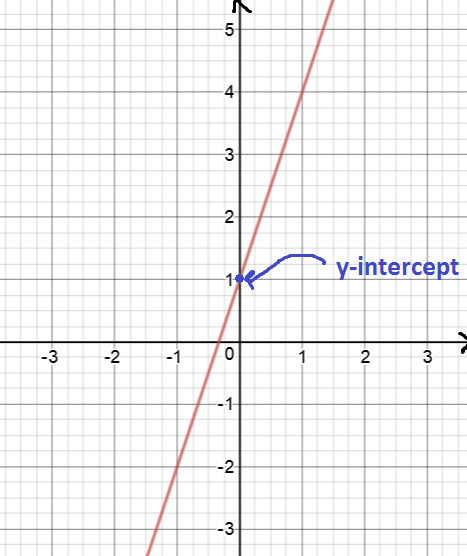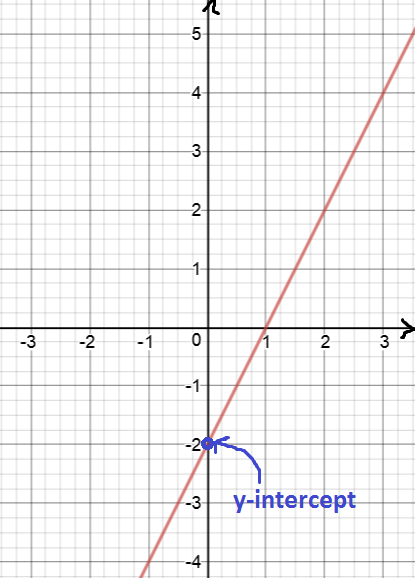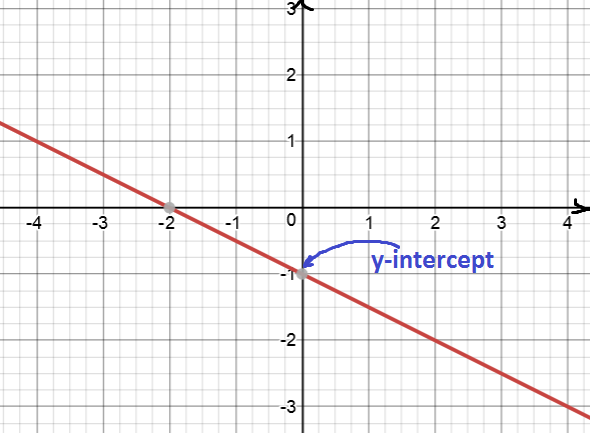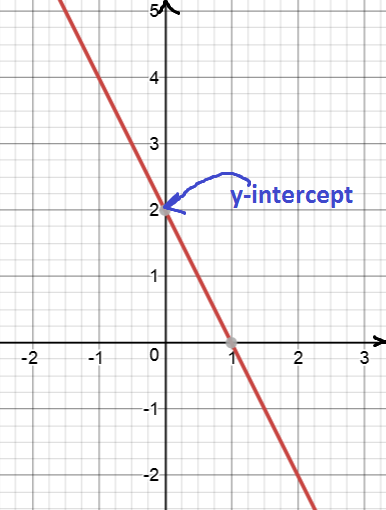Pull Down & Release to Refresh

# What is the y-intercept of a Straight Line

The $y$-intercept of a straight line is the point where the line crosses the $y$-axis. In other words, it's the point on the line where the $x$-value is zero.

Let's have a look at a few examples of straight lines and their $y$-intercepts.

## Example 1In this graph, the line crosses the $y$-axis at $y = 1$. So, the $y$-intercept is $1$.

## Example 2In this graph, the line crosses the $y$-axis at $y = -2$. So, the $y$-intercept is $-2$.

## Example 3In this graph, the line crosses the $y$-axis at $y = -1$. So, the $y$-intercept is $-1$.

## Example 4In this graph, the line crosses the $y$-axis at $y = 2$. So, the $y$-intercept is $2$.

### Description

coordinate geometry is a branch of geometry where the position of the points on the plane is defined with the help of an ordered pair of numbers also known as coordinates. In this tutorial series, you will learn about vast range of topics such as Cartesian Coordinates, Midpoint of a Line Segment etc

### Audience

year 10 or higher, several chapters suitable for Year 8+ students.

### Learning Objectives

Explore topics related to Coordinates Geometry

Author: Subject Coach
You must be logged in as Student to ask a Question.